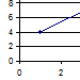# Data points needed for Universal Scalability Curves

I did some testing for a single transaction type in to a SOA environment. For different numbers of threads (workload) in Jmeter I measured the throughput (transaction per second) for a 10 minute period. The results are from the graph below:From looking at the results I wondered how well I could apply Neil Gunther’s
(Universal Scalability Law). The USL is an equation that allows you to take a sparse set of load measurements and from those determine how your application will scale under larger user loads than you may be able to generate in your test lab. This can all be done in a spreadsheet tool like Excel.

I was interested to see just how many data points I would need. So, I plugged my data into Excel. I did three predictions. The first was using all the 8 data points collected during the test. Next I used the first 4 collected during the test and finally I used 4 data points spread throughout the test. The graph below shows the predicted scalability curves.Using the first 4 data points (which looked linear’ish) then it predicted the maximum throughput would be around 75 tps and the graph didn’t show the degradation at higher thread values. What was interesting was that the spreadout results are close to the predicted curve using all the measurements.

This was my first attempt to use the scalability curve. The spreadsheet from Neil website was easy to use and I am impressed in this example that with only a few datapoints the results were close to the prediction curve using all the data points. I think I will need to do more similar experiments before I am a convert! But it looks promising.